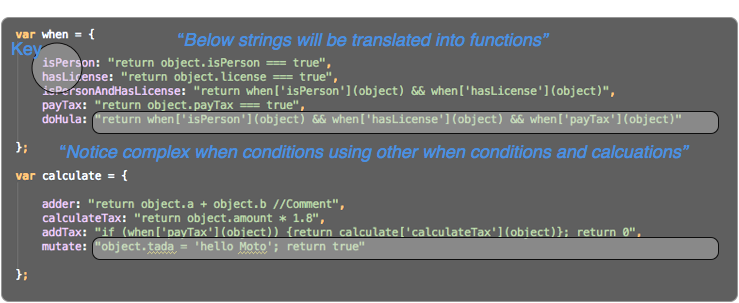# npm

## rules-when

0.0.5 • Public • Published

# rules-when

Simple When Rule Construction

[W I P Initial Feb 18th, 2015]

## BizRez When Rules

A simple way to dynamically create when rules - A great example how to use the new Function instead of the 'evil' eval.## Intro

Simplicity is elegance.

What we need is a descriptive way to tag Biz(i)ness Rules and catalog, inventory, usage, and change dynamically. Javascript provides a simple way of dynamically creating functions on the fly.

Prime directives, and ables ...

• Easy to understand the code
• Thin
• Self documenting
• Pattern - not an enforcement
• Crazy fast
• Secure from XXS

## Dependencies

• Mocha.js for for test infecting the code (good infection)

## A few Use Cases for Usage

• Validations
• Calculations
• Automated business rules - decision engine
• Home security, sprinkler systems, and darn maybe your car...

## Benefits

• Repository of rules
• Traceability
• Modify the rule not the code
• Dynamic changeability - conditions change so do the execution
• Easy to understand and modify
• Extensible
• Maintainable
• Reusable
• Chainable
• Micro foot print and scalable

## Example Setup

``````
//Example Setup

var when = {

isPerson: "return object.isPerson === true //Comment",
payTax: "return object.payTax === true",
doHula: "return when['isPerson'](object) && when['hasLicense'](object) && when['payTax'](object)"

}

var calculate = {

adder: "return object.a + object.b //Comment",
calculateTax: "return object.amount * 1.8",
addTax: "if (when['payTax'](object)) {return calculate['calculateTax'](object)}; return 0",
mutate: "object.tada = 'hello Moto'; return true"

}

//Describe Decision Table
var decisionTable = {

calcNumber: [["return when['isPerson'](object)", "return calculate['adder'](object)", "return true"],
["return when['isPerson'](object)", "return calculate['calculateTax'](object)", "return true"],
["return when['isPerson'](object)", "return calculate['calculateTax'](object)", "return true"]
]

}

``````

### image 1

Notice: functions stored as strings. These strings will be translated via the new Function operation### Translate value into functions

``````        //Create When Condtion functions

//Convert key[value] into real functions
for (var key in when) {
when[key] = new Function('object', 'when', 'calculate', when[key]);
}

}

//Create Calculation functions
//Convert key[value] into real functions
for (var key in calculate) {
calculate[key] = new Function('object', 'when', 'calculate', calculate[key]);

}
}

``````

## Example Run

``````rules.loadWhenRules(when);

var person = {isPerson: true, license: true, a: 5, b: 10, amount: 500, payTax: true};

console.log("isPerson:" + rules.when('isPerson', person));
rules.calculate('mutate',person);
console.log("decisiontable result:" + rules.decisionTable('calcNumber', person));
console.log("doHula:" + rules.when('doHula',person));

``````

mocha ./lib/test

## Keywords

### Install

`npm i rules-when`

### Repository

github.com/mallond/rules-when

### Homepage

github.com/mallond/rules-when